# Eigenvalues and eigenvectors facts for kids

Kids Encyclopedia Facts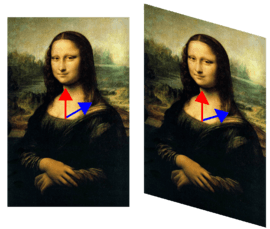Illustration of a transformation (of Mona Lisa): The image is changed in such a way that the red arrow (vector) does not change its direction, but the blue one does. The red vector therefore is an eigenvector of this transformation, the blue one is not. Since the red vector does not change its length, its eigenvalue is 1. The transformation used is called shear mapping.

Linear algebra talks about types of functions called transformations. In that context, an eigenvector is a vector—different from the null vector—which does not change direction after the transformation (except if the transformation turns the vector to the opposite direction). The vector may change its length, or become zero ("null"). The eigenvalue is the value of the vector's change in length, and is typically denoted by the symbol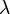$\lambda$. The word "eigen" is a German word, which means "own" or "typical".

## Basics

If there exists a square matrix called A, a scalar λ, and a non-zero vector v, then λ is the eigenvalue and v is the eigenvector if the following equation is satisfied: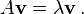$A\mathbf{v} = \lambda \mathbf{v} \, .$

In other words, if matrix A times the vector v is equal to the scalar λ times the vector v, then λ is the eigenvalue of v, where v is the eigenvector.

An eigenspace of A is the set of all eigenvectors with the same eigenvalue together with the zero vector. However, the zero vector is not an eigenvector.

These ideas often are extended to more general situations, where scalars are elements of any field, vectors are elements of any vector space, and linear transformations may or may not be represented by matrix multiplication. For example, instead of real numbers, scalars may be complex numbers; instead of arrows, vectors may be functions or frequencies; instead of matrix multiplication, linear transformations may be operators such as the derivative from calculus. These are only a few of countless examples where eigenvectors and eigenvalues are important.

In cases like these, the idea of direction loses its ordinary meaning, and has a more abstract definition instead. But even in this case, if that abstract direction is unchanged by a given linear transformation, the prefix "eigen" is used, as in eigenfunction, eigenmode, eigenface, eigenstate, and eigenfrequency.

Eigenvalues and eigenvectors have many applications in both pure and applied mathematics. They are used in matrix factorization, quantum mechanics, facial recognition systems, and many other areas.

## Example

For the matrix A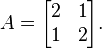$A = \begin{bmatrix} 2 & 1\\1 & 2 \end{bmatrix}.$

the vector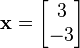$\mathbf x = \begin{bmatrix} 3 \\ -3 \end{bmatrix}$

is an eigenvector with eigenvalue 1. Indeed, one can verify that: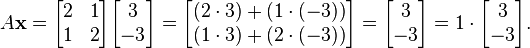$A \mathbf x = \begin{bmatrix} 2 & 1\\1 & 2 \end{bmatrix} \begin{bmatrix} 3 \\ -3 \end{bmatrix} = \begin{bmatrix} (2 \cdot 3) + (1 \cdot (-3)) \\ (1 \cdot 3) + (2 \cdot (-3)) \end{bmatrix} = \begin{bmatrix} 3 \\ -3 \end{bmatrix} = 1 \cdot \begin{bmatrix} 3 \\ -3 \end{bmatrix}.$

On the other hand the vector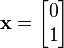$\mathbf x = \begin{bmatrix} 0 \\ 1 \end{bmatrix}$

is not an eigenvector, since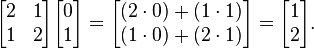$\begin{bmatrix} 2 & 1\\1 & 2 \end{bmatrix} \begin{bmatrix} 0 \\ 1 \end{bmatrix} = \begin{bmatrix} (2 \cdot 0) + (1 \cdot 1) \\ (1 \cdot 0) + (2 \cdot 1) \end{bmatrix} = \begin{bmatrix} 1 \\ 2 \end{bmatrix}.$

and this vector is not a multiple of the original vector x.

## Related pages

• .
• .
• .
• .
• .
• .
• .
• .
• .
• .
• . ISBN: 0-521-30586-1 (hardback), ISBN: 0-521-38632-2 (paperback)
• .
• .
• .
• .
• .
• .
• .
• .
• .
• .
• .
• .
• .
• .
• .
• .
• Pigolkina, T. S. and Shulman, V. S., Eigenvalue (in Russian), In:Vinogradov, I. M. (Ed.), Mathematical Encyclopedia, Vol. 5, Soviet Encyclopedia, Moscow, 1977.
• .
• .
• Curtis, Charles W., Linear Algebra: An Introductory Approach, 347 p., Springer; 4th ed. 1984. Corr. 7th printing edition (August 19, 1999), ISBN: 0-387-90992-3.
• .
• .
• .
Theory
Online calculators

## Images for kidsEigenvalues and eigenvectors Facts for Kids. Kiddle Encyclopedia.